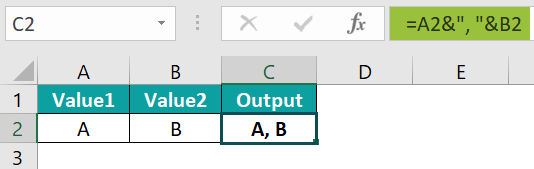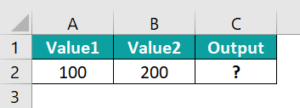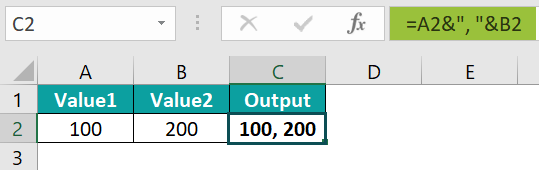# Combine Cells In ExcelArticle byExcelMojo Team## What Is Combine Excel Cells?

Combine Cells in Excel allows users to join two or more cells while keeping their content intact. It is especially useful when dealing with data sets that require consolidating values, such as financial statements or inventory lists.

For example, the below table has two values in Excel. Now, using Text Strings with the Ampersand, let us learn how to combine the cells.

The steps to combine cells are:

1. Choose the cell where we will enter the formula. For this illustration, let us select cell C2.

2. Enter the Ampersand formula =A2& “,” &B2 into cell C2.

3. We can see the result as shown in the below image.###### Key Takeaways
• Combining cells allows you to retain crucial data relationships while presenting them in a sleek, visual format essential for making informed decisions based on your data-driven insights.
• Combining cells streamlines workflow and enhances accessibility in tasks like creating reports or financial statements, allowing one to take their work organization and productivity skills to new heights.
• Excel’s “Combine Cells” feature allows users to merge two or more cell values into a cohesive unit.
• This feature is particularly useful when combining first and last names or entering location details with name information.

### How To Combine Cells In Excel?

We can combine cells in excel in many ways. The most common approach involves merging cells in excel, where two or more adjacent cells are joined into a single cell while retaining the data. This can be done by selecting the target cells, clicking on the Merge & Center or Merge Across option under the Home tab, and choosing an alignment option if desired.

Another method uses Excel’s formula feature to concatenate text from multiple cells into a new cell. To do this, users must write a formula that specifies which cells should be combined and how they should be separated – typically with commas or spaces.

### Examples

#### Example #1 – Combine A Text String And A Number With The Ampersand Operator

The following example combines text string and number, in columns A and B with the ampersand operator.

The steps are:

• Step 1: Select the cell where we enter the formula. For this example, let us choose cell C2.
• Step 2: Input the complete formula =A2& “,” &B2 into cell C2.
• Step 3: Press Enter key. We can see the combined value in cell C2.

Now, press Enter and drag the cursor to cell C6 to obtain results in other cells.

#### Example #2 – Combine Two Text Strings With The Ampersand And CONCATENATE

The following example shows two values in Excel. Now, let us combine two text strings with the Ampersand and CONCATENATE functions in excel.

The steps are:

• Step 1: Select the cell where we will enter the formula. For this example, let us choose cell C2.
• Step 2: Enter the Ampersand formula =A2& “ ” &B2 into cell C2.
• Step 3: Press the Enter key. We can see the result in cell C2.

Now, press Enter and drag the cursor to cell C6 to obtain results.

• Step 4: Now, enter the CONCATENATE formula =CONCATENATE (A2,“ ”,B2) into cell E2.
• Step 5: Press the Enter key. We can see the result in cell E2.

Now, press Enter and drag the cursor to cell E6 to obtain results.

#### Example #3 – Combine Two Text Strings And Add A Line Break With The Ampersand And CHAR

The following table has two values. Now, let us learn how to combine two text strings and adding a line break with the ampersand and CHAR function in excel.

The steps are:

• Step 1: Select the cell where we will enter the formula. For this example, let us choose cell C2.
• Step 2: Input the complete formula =A2&CHAR (10) &B2 into cell C2.
• Step 3: Press Enter key. We can see the result in cell C2. Now, press Enter and drag the cursor to cell C3 to obtain the result.

#### Example #4 – Prefix And Suffix Text Strings To A Number With The Ampersand

The following example has 2 values in Excel. Now, let us learn how to combine cells using the Prefix and Suffix Text Strings to a Number with the Ampersand.

The steps are:

• Step 1: Select the cell where we will enter the formula. For this example, let us choose cell C2.
• Step 2: Input the Ampersand formula =A2& “,” &B2 into cell C2.

#### Example #5 – Prefix Text Strings To The Current Date With Ampersand

The following table has 2 values in cells A2 and A3. Now, let us combine two values in Excel with Prefix Text Strings and Ampersand.

The steps are:

• Step 1: Select the cell where we will enter the formula. For this example, let us choose cells A2 & A3.
• Step 2: Input the Complete formula

=“Today is “&TODAY() into cell A2

=”Today is “&TEXT(TODAY(),”mm-dd-yyyy”) into cell A3.

• Step 3: Press the Enter key.

We can see the results as shown in the below image.

#### Example #6 – Combine Cell Values In VBA With The Ampersand

We require a VBA code to merge the values of cells A4 and B4 into a single Excel cell, C4. The following code will combine the cell values without any data loss:

Sub MergeCells()
Range(“C4”). Value = Range(“A4”). Value & Range(“B4”). Value
End Sub

Execute the above VBA code in the VBA Editor to achieve the desired output. This code will effectively merge the values of cells A4 and B4 into cell C4.

### Important Things To Note

• When using this feature, users can select the cells they want to combine and choose how they want the content to appear in the merged cell.
• This function also has options for adding separators between merged cells or leaving extra spaces between them as per user preference.
• The Combine Cells function saves time and eliminates errors by making data more organized and readable.
• There are several methods for combining Excel cells, including the use of the ampersand (&), Concatenate (), Char (), Text (), and VBA Code. Each method offers unique benefits and can be tailored to suit individual needs.
• One particularly useful function is the TODAY function in excel, which automatically returns the current date each time the workbook is opened. This function requires no arguments and is a simple yet effective way to keep track of important dates.

1. Why would I need to combine cells in Excel?

Combining cells in Excel allows users to merge multiple cells into a single, larger cell, often to make data easier to understand or more visually appealing. We can also create headers or titles spanning several columns.
The following example has two values in cell A2 and B2. Now, let us understand how to employ Numerical values with the Ampersand.The steps are:

• Step 1: Select cell C2.

• Step 2: Input the Ampersand formula =A2& “,” &B2 into cell C2.

• Step 3: We can see the result in cell C2.2. What are some formatting options for combined cells in Excel?

The most commonly used option is Merge and Center, which combines the selected cells and aligns the text to the center of the newly created cell. It decreases the readability of data but is useful for creating titles or headings for our worksheet.
• Wrap text in in exc in excel expands the width of the combined cells to fit every character in them while keeping them on a single line.
Additionally, we can split one big cell into several small ones using Text-to-Columns. This allows to separate contents based on special characters, spaces, and other delimiters between them.

3. Are there any limitations to the number of cells that can be combined?

One limitation is the power output, which increases with the number of cells. If too many cells are connected in series, there is a risk of exceeding the voltage rating of the battery or causing damage to other components.
Additionally, connecting too many cells in parallel can result in imbalanced charging and discharging rates, leading to decreased efficiency and reduced overall lifespan of the battery.